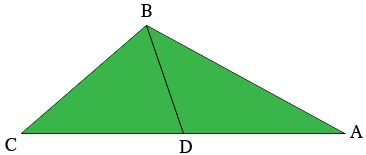SEARCH HOMEMath Central Quandaries & QueriesQuestion from Yoonji: the 3 sides of a triangular garden measure 200 ft., 250 ft., and 300 ft., respectively. the garden is to be divided by a line bisecting the longest side and drawn from the opposite vertex. what is the length of the bisecting line?Hi,

I drew a rough sketch of the garden with the bisecting line.$|AB| = 250$ feet, $BC| = 200$ feet, $|CA| = 300$ feet and $D$ is the midpoint of $CA.$

In problems involving triangles you quite often have to use the Law of Sines or the Law of Cosines. Looking at the triangle $ABD,$ you know the lengths of $AB$ and $DA$ and hence if you knew the measure of the angle $DAB$ you could use the Law of Cosines to find the length of $BD.$ Can you use what you know about the triangle $ABC$ to determine the measure of the angle $DAB?$

PennyMath Central is supported by the University of Regina and The Pacific Institute for the Mathematical Sciences.